NEET  >  Sample AFMC Physics Mock Test

# Sample AFMC Physics Mock Test

Test Description

## 50 Questions MCQ Test AFMC Mock Test Series | Sample AFMC Physics Mock Test

Sample AFMC Physics Mock Test for NEET 2023 is part of AFMC Mock Test Series preparation. The Sample AFMC Physics Mock Test questions and answers have been prepared according to the NEET exam syllabus.The Sample AFMC Physics Mock Test MCQs are made for NEET 2023 Exam. Find important definitions, questions, notes, meanings, examples, exercises, MCQs and online tests for Sample AFMC Physics Mock Test below.
Solutions of Sample AFMC Physics Mock Test questions in English are available as part of our AFMC Mock Test Series for NEET & Sample AFMC Physics Mock Test solutions in Hindi for AFMC Mock Test Series course. Download more important topics, notes, lectures and mock test series for NEET Exam by signing up for free. Attempt Sample AFMC Physics Mock Test | 50 questions in 30 minutes | Mock test for NEET preparation | Free important questions MCQ to study AFMC Mock Test Series for NEET Exam | Download free PDF with solutions
 1 Crore+ students have signed up on EduRev. Have you?
Sample AFMC Physics Mock Test - Question 1

### Best neutron moderator is

Detailed Solution for Sample AFMC Physics Mock Test - Question 1

Heavy Water is an excellent moderator due to its high moderating ratio and low absorption cross section for neutrons.

Sample AFMC Physics Mock Test - Question 2

### Mass defect of an atom refers to

Detailed Solution for Sample AFMC Physics Mock Test - Question 2

A mass defect refers to the difference in mass between of an atom and the sum of the masses of the protons, neutrons, and electrons of the atom. The "missing" mass is the energy released by the formation of the atomic nucleus.

Sample AFMC Physics Mock Test - Question 3

### Who indirectly determined the mass of the electron by measuring the charge of the electron ?

Detailed Solution for Sample AFMC Physics Mock Test - Question 3

Millikan determined the mass of the electron by measuring the charge of the electron.

Sample AFMC Physics Mock Test - Question 4

Resistance of a wire at 20°C is 20 Ω and at 500°C is 60 Ω. At what temperature its resistance is 25 Ω ?

Detailed Solution for Sample AFMC Physics Mock Test - Question 4

RT=60 ohms @ T=500oC

Also, RT′​=20 ohms @ T′=20oC

Resistance at a given temperature RT​=RT′​[1+α(T−T′)]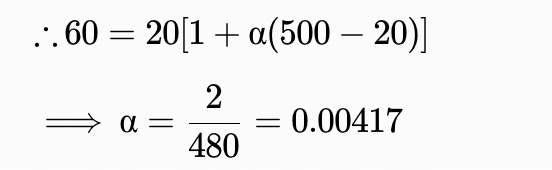Let resistance of wire is 25 ohm at temperature T′′.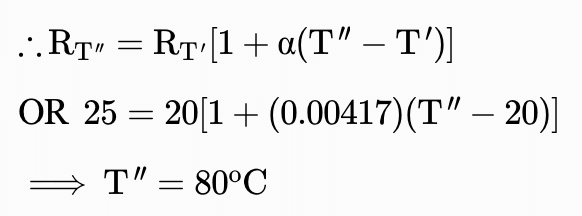Sample AFMC Physics Mock Test - Question 5

Photo cell is a device to

Detailed Solution for Sample AFMC Physics Mock Test - Question 5

Photo cell is used to convert photon energy(i.e. light energy) into chemical energy.

Sample AFMC Physics Mock Test - Question 6

A nichrome wire 50cm long and square millimeter cross-section carries a current of 4A when connected to a 2V battery. The resistivity of nichrome wire in ohm meter is

Detailed Solution for Sample AFMC Physics Mock Test - Question 6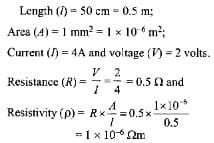Sample AFMC Physics Mock Test - Question 7

Two coils are placed close to eachother. The mutual inductance of the pair of coils depends upon

Detailed Solution for Sample AFMC Physics Mock Test - Question 7

The coupled flux of two coils system is used to define the mutual inductance between the coils. The mutual inductance between the coils is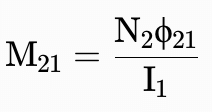So it is defined as the proportionality between the emf generated in coil 2 due to the current flows in coil 1. Thus It depends on the relative position and orientation of two coils.

Sample AFMC Physics Mock Test - Question 8

The p.d. across an instrument in an A.C. circuit of frequency f is V and the current flowing through it is I such that V = 5 cos 2π amp. The power dissipated in the instrument is

Detailed Solution for Sample AFMC Physics Mock Test - Question 8

V = 5cos2πft    [ω = 2πf]

V = 5cosωt

=5sin(ωt+π2)  and i = 2sinωt

Power = Vrms×irms×cosφ=0

(Since φ = π/2, therefore cosφ = cos(π/2)=0)

Sample AFMC Physics Mock Test - Question 9

The current flowing in a coil of self inductance 0.4 mH is increased by 250 mA in 0.1 s. The e.m.f. induced will be

Detailed Solution for Sample AFMC Physics Mock Test - Question 9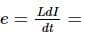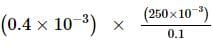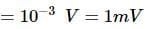As current is increasing, induced e.m.f. is in opposite direction, therefore, e = - 1 mV.

Sample AFMC Physics Mock Test - Question 10

The electrostatic capacitance depends on

Detailed Solution for Sample AFMC Physics Mock Test - Question 10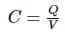The capacitance is proportional to the surface area of the conducting plate and inversely proportional to the distance between the plates. Hence, it depends upon the size of the conductor, and not upon the nature of conductor, thickness or colour.

Sample AFMC Physics Mock Test - Question 11

If size of the earth is reduced to half, then no. of days in one year will be

Detailed Solution for Sample AFMC Physics Mock Test - Question 11

Time period is not dependent on radius.

Sample AFMC Physics Mock Test - Question 12

The capacity of a condenser with two identical plates separated by a distance 'd' in air is 'C'. If the distance of separation is reduced to d/2, the capacity is

Detailed Solution for Sample AFMC Physics Mock Test - Question 12

The capacity of a parallel plate condenser is given as,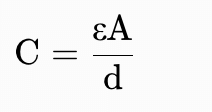When the separation between the plates is halved then the new capacity of the condenser is given as,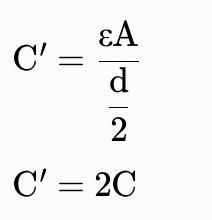Thus, the new capacity of parallel plate condenser is 2C.

Sample AFMC Physics Mock Test - Question 13

Figure shows two large cylindrical shells having uniform linear charge densities + λ and - λ . Radius of inner aylinder is 'a' and that of outer cylinder is 'b'. A charged particle of mass m , charge q revolves in a circle of radius r. Then its speed 'V' is:
[Neglect gravity and assume the radii of both cylinders to be very small in comparison to their length]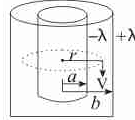Detailed Solution for Sample AFMC Physics Mock Test - Question 13

Using Gauss's law for electrostatics,

Electric field at charged particle would be given as,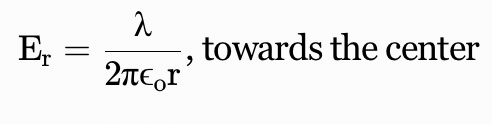So centripetal force on particle is,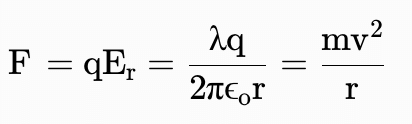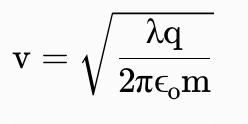Sample AFMC Physics Mock Test - Question 14

The energy emitted per second by a black body at 27°C is 10 J. If the temperature of the black body is increased to 327°C, the energy emitted per second will be

Detailed Solution for Sample AFMC Physics Mock Test - Question 14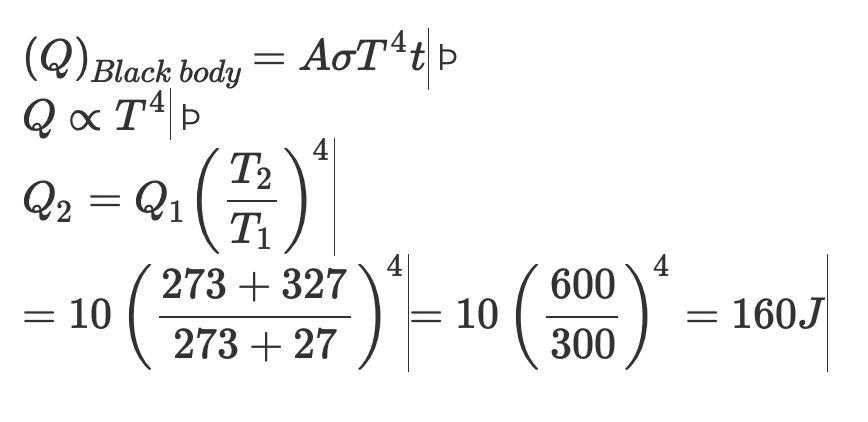Sample AFMC Physics Mock Test - Question 15

Vapour is injected at a uniform rate in a closed vessel which was initially evacuated. The pressure in the vessel

Detailed Solution for Sample AFMC Physics Mock Test - Question 15

When the vapour is injected into the evacuated vessel, the pressure of the vessel starts to increase. As the pressure is increased and reaches the saturated vapour pressure, the vapour starts to condense beyond this. Therefore, after a particular instant, the pressure of the container becomes constant.

So, the pressure increases initially and then becomes constant.

Sample AFMC Physics Mock Test - Question 16

At what temperature, the root mean square velocity of a hydrogen molecules is equal to that of an oxygen molecules at 47°C?

Detailed Solution for Sample AFMC Physics Mock Test - Question 16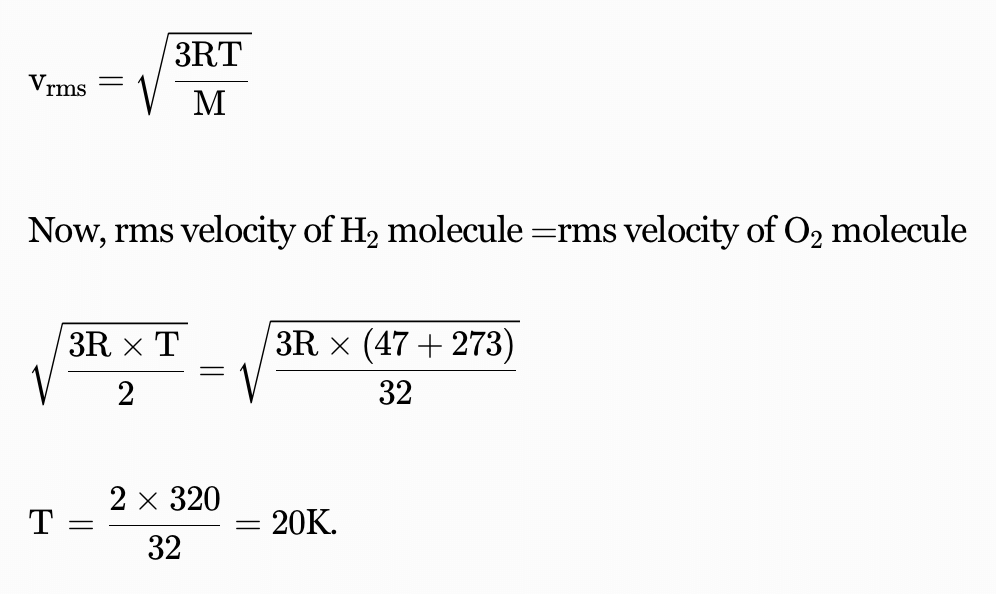Sample AFMC Physics Mock Test - Question 17

The diameter of a copper sphere is 6 cm. It is melted to form a wire of uniform cross-section. If the length of the wire is 36 cm then find the radius of the wire.

Detailed Solution for Sample AFMC Physics Mock Test - Question 17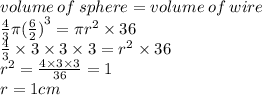Sample AFMC Physics Mock Test - Question 18

If a long hollow copper pipe carries a current, the produced magnetic field will be

Detailed Solution for Sample AFMC Physics Mock Test - Question 18

If the current flows on the surface of a long hollow copper pipe. Then the magnetic field inside is zero. Hence, there shall be magnetic field produced outside the pipe.

Sample AFMC Physics Mock Test - Question 19

A ball of mass m strikes elastically a wall at an angle of 30° with velocity ν. The change in momentum is

Detailed Solution for Sample AFMC Physics Mock Test - Question 19

Change in momentum (P)=2mv cosθ

P=2mv cos 30°

P=2mv√3/2

P=√3mv

Sample AFMC Physics Mock Test - Question 20

In a surface tension experiment with a capillary tube, water rises upto 8 mm. If the same experiment is repeated on an artificial satellite, which is revoling around the earth, water will tise in capillary tube upto a height of

Detailed Solution for Sample AFMC Physics Mock Test - Question 20
• Height of capillary rise liquid is given by h = 2T/(rρg) . T is surface tension, r is radius of capillary tube, ρ is density and g is acceleration due to gravity.
• Since acceleration due to gravity is in denominator and acceleration due to gravity is negligibly small at the orbit where satteleite is revolving around the earth, capillary rise will be very high.
• So 'full length' may be the correct answer.
Sample AFMC Physics Mock Test - Question 21

Two solid pieces, one of steel and the other of aluminium when immersed completely in water have equal weights. When the solid pieces are weighed in air

Detailed Solution for Sample AFMC Physics Mock Test - Question 21

Apparent weight in air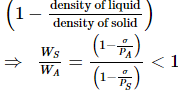Sample AFMC Physics Mock Test - Question 22

If pressure inside two soap bubbles are 1.01 and 1.02 atm respectively, then the ratio between their volumes is (Take atmospheric pressure = 1 atm)

Detailed Solution for Sample AFMC Physics Mock Test - Question 22

Pressure × Radius = Surface Tension
Here the pressure is Gauge Pressure, i.e the difference between absolute pressure and atmospheric pressure
Hence ratio of gauge pressures is 1:2
Accordingly ratio of radii is 2:1
Hence ratio of volumes is 8:1

Sample AFMC Physics Mock Test - Question 23

An incompressible fluid flows steadily through a cylindrical pipe which has radius 2 R at point A and radius R at point B. If velocity at point A is ν, then its velocity at point B is

Detailed Solution for Sample AFMC Physics Mock Test - Question 23

ATQ, equation of continuity,

Area is inversely proportional to flow rate,

therefore if area is reduced by four times

the flow rate becomes 4 times = 4v

Sample AFMC Physics Mock Test - Question 24

The potential energy of a molecule on the surface of a liquid compared to one inside the liquid is

Detailed Solution for Sample AFMC Physics Mock Test - Question 24
• When the surface area of  the liquid is increased molecules from the interior of the liquid rise to surface.
• As these molecules reach the surface work is done against the cohesive force.This work is stored in the molecules in the form of potential energy.
• Thus, the  potential energy of the molecules lying in the surface is greater than that of the molecules in the interior of the liquid.
Sample AFMC Physics Mock Test - Question 25

Water level rises in a capilary tube, if the angle of contact is

Detailed Solution for Sample AFMC Physics Mock Test - Question 25

If the angle of contact is less than 90 ,then the level of water rises in concave meniscus.

Sample AFMC Physics Mock Test - Question 26

Which of the following affets the elasticity of a substance?

Detailed Solution for Sample AFMC Physics Mock Test - Question 26
• Elasticity depends on temperature.
• Also, elasticity changes due to impurities because they increase binding of crystal grains., hence enhancing elasticity.
• Hammering also changes the structure of material as crystal grains break up into smaller units which plays a large role in determining elasticity (increases elasticity).
• Also, annealing tends to form a uniform orientation of crystal grains, hence producing larger crystal and changing elasticity.
Sample AFMC Physics Mock Test - Question 27

A wire can be broken by applying a load of 200 N.The force required to break another wire of the same length and same material, but double in diameter, is

Detailed Solution for Sample AFMC Physics Mock Test - Question 27

We know that, E = -dΦ/dt,
E = -d/dt {2t² - 3t + 1}
= -(4t - 3)
at t = 0.25 sec,...
E = -(4×0.25 - 3) = 2 V

Sample AFMC Physics Mock Test - Question 28

A body starts from rest. What is the ratio of the distance travelled by the body during the 4th and 3rd second

Detailed Solution for Sample AFMC Physics Mock Test - Question 28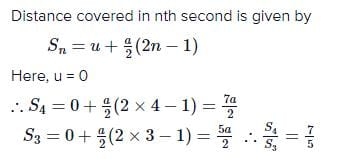Sample AFMC Physics Mock Test - Question 29

For a projectile, the ratio of maximum height reached to the square of flight time is (g = 10 ms⁻2)

Detailed Solution for Sample AFMC Physics Mock Test - Question 29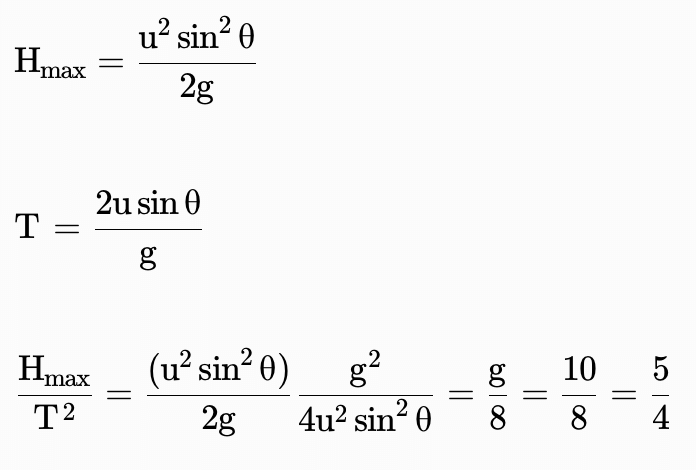Sample AFMC Physics Mock Test - Question 30

To increase the magnification of a telescope

Detailed Solution for Sample AFMC Physics Mock Test - Question 30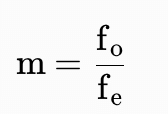From the above relation we can see that magnification is directly proportional to the focal length of objective lens.

Sample AFMC Physics Mock Test - Question 31

The potential energy of a particle executing simple harmonic motion at a distance y from its equilibrium position is

Detailed Solution for Sample AFMC Physics Mock Test - Question 31

Potential Energy =  1/2 k y2

ω= k/m

k = mω2

Potential Energy = 1/2 (mω) y2

Potential Energy = 1/2 mω2y2

Sample AFMC Physics Mock Test - Question 32

The frequency of a light wave in a material is 2 x 1014 H z and wavelength is 5000 Å. The refractive index of material will be

Detailed Solution for Sample AFMC Physics Mock Test - Question 32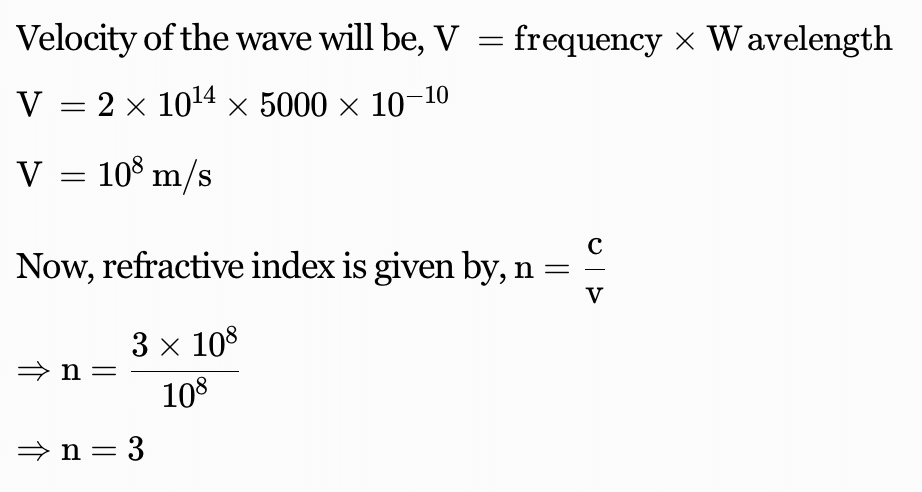Sample AFMC Physics Mock Test - Question 33

Time of exposure for a photographic print is 10 second, when a lamp of 50 Cd is placed at 1 m from it. Then another lamp of luminous intensity I is used, and is kept at 2 m from it. If the time of exposure now is 20 s, the value of I in (in Cd) is :

Detailed Solution for Sample AFMC Physics Mock Test - Question 33

Intensity ∝ time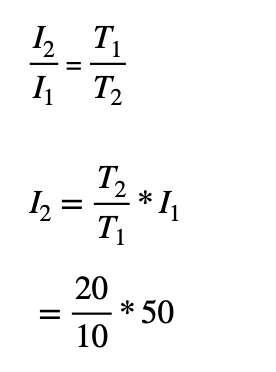= 100 Cd

Sample AFMC Physics Mock Test - Question 34

When a sphere of moment of inertia I about an axis through its centre of gravity and mass m rolls down from rest, on an inclined plane without slipping, its kinetic energy is

Detailed Solution for Sample AFMC Physics Mock Test - Question 34

Rolling motion= Pure translatory motion + Pure rotatory motion

Rolling motion= 1/2 mν2+1/2Iω2

Sample AFMC Physics Mock Test - Question 35

When light travels from one medium to another which are separated by a sharp boundary, the characteristic of light which does not change, is

Detailed Solution for Sample AFMC Physics Mock Test - Question 35

Color and energy depend on frequency and frequency does not change. The light photons are the same as the photons at the beginning. Frequency is a parameter of the source of emission of light, not the medium it is travelling through.

Sample AFMC Physics Mock Test - Question 36

A wheel is rotating at 900 r.p.m. about its axis When power is cut off it comes to rest in 1 minute The angular retardation in rad/s2 is

Detailed Solution for Sample AFMC Physics Mock Test - Question 36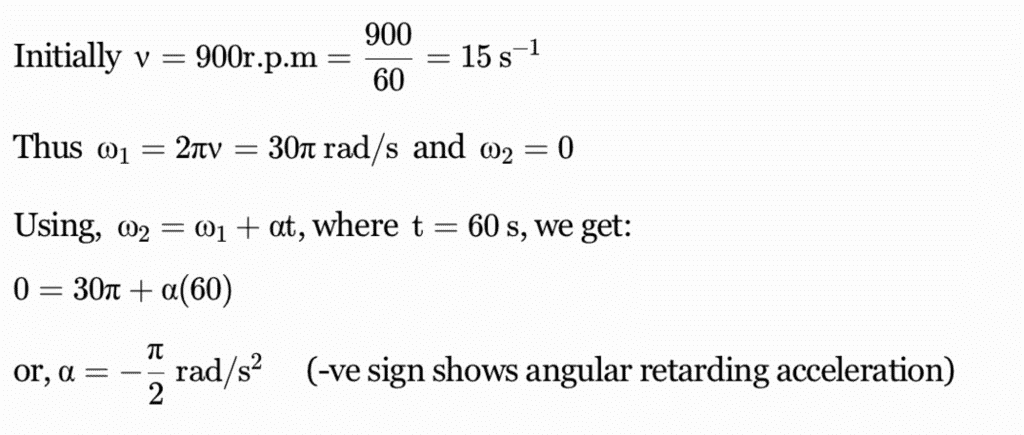Sample AFMC Physics Mock Test - Question 37

A body is rotating with angular velocity 30 rad/s. If its kinetic energy is 360 J, then its moment of inertia is

Detailed Solution for Sample AFMC Physics Mock Test - Question 37

Kinetic Energy(KE) = 360J

Angular Speed = ω = 30rad/sec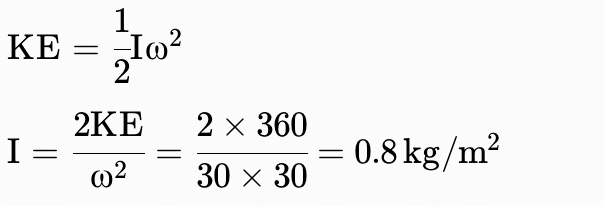Sample AFMC Physics Mock Test - Question 38

A ballet dancer of moment of inertia I is spinning about the vertical axis with an angular velocity of 20 rad-s⁻1. If she suddenly changes her angular velocity to 10 rad-s⁻1, then new moment of inertia would be

Detailed Solution for Sample AFMC Physics Mock Test - Question 38

Here, angular momentum is conserved.

Initial angular momentum = Final angular momentum

I×20=I′×10

where, I′ is the new moment of inertia.

Then,

I′= 2 I

Sample AFMC Physics Mock Test - Question 39

A fan is making 600 rev-min⁻1. If it makes 1200 rev-m⁻1, then increase in its angular velocity is

Detailed Solution for Sample AFMC Physics Mock Test - Question 39

Initial angular velocity= ω= 2πn1

Final angular velocity= ω2​ = 2πn2

Increment in angular velocity ω = 2π(n−n1)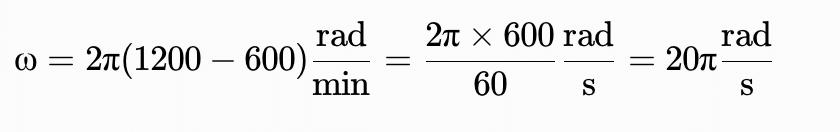Sample AFMC Physics Mock Test - Question 40

The period of spring oscillating simple harmonically

Detailed Solution for Sample AFMC Physics Mock Test - Question 40

The period of a simple harmonic oscillator is given by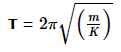Sample AFMC Physics Mock Test - Question 41

A p-type semiconductor is

Detailed Solution for Sample AFMC Physics Mock Test - Question 41

Any type of semiconductor either p or n type both are neutral i.e. uncharged.

Sample AFMC Physics Mock Test - Question 42

At 27°C a gas is suddenly compressed such that its pressure becomes 1/8th of original pressure. Temperature of the gas will be (γ = 5/3)

Detailed Solution for Sample AFMC Physics Mock Test - Question 42

Since gas is compressed suddenly, gas is undergone an adiabatic process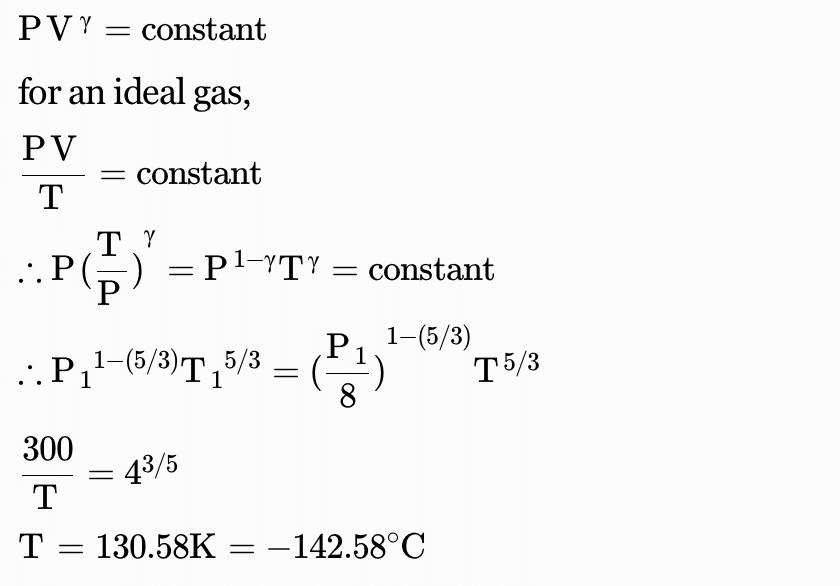Sample AFMC Physics Mock Test - Question 43

The length and breath of a metal sheet are 3.124 m and 3.002 m respectively. The area of this sheet up to four significant figures is

Detailed Solution for Sample AFMC Physics Mock Test - Question 43

Area of metal sheet =3.124×3.002 = 9.378248

Now, the result must have significant figures equal to the least of figure being multiplied.

So, area of metal sheet =9.378 m2

Sample AFMC Physics Mock Test - Question 44

SI unit of pressure is

Detailed Solution for Sample AFMC Physics Mock Test - Question 44

The SI unit for pressure is the pascal (Pa), equal to one newton per square metre.

Sample AFMC Physics Mock Test - Question 45

A suitable unit for gravitational constant is

Detailed Solution for Sample AFMC Physics Mock Test - Question 45

The gravitational force is given as: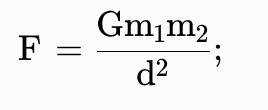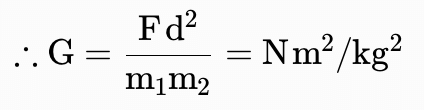Sample AFMC Physics Mock Test - Question 46

If the two slits in Young's experiment have width ratio 1:4, the ratio of intensity at maxima and minima in the interference pattern in

Detailed Solution for Sample AFMC Physics Mock Test - Question 46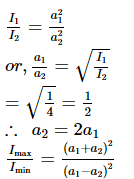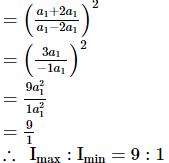Sample AFMC Physics Mock Test - Question 47

When a source is linear, the wavefront is

Detailed Solution for Sample AFMC Physics Mock Test - Question 47

When the source of disturbance is a slit (i.e. line source) then the wavefront is cylindrical because all the points are equidistant from the source and they lie on the surface of the cylinder.

Sample AFMC Physics Mock Test - Question 48

According to wave theory, light is a form of energy which travels through a medium in the form of

Detailed Solution for Sample AFMC Physics Mock Test - Question 48
• Light is an example of a transverse wave.
• For transverse waves in matter, the displacement of the medium is perpendicular to the direction of propagation of the wave.
Sample AFMC Physics Mock Test - Question 49

Standing waves are produced in a 10 m long stretched string. If the string vibrates in 5 segments and the wave velocity is 20 m/s, the frequency is

Detailed Solution for Sample AFMC Physics Mock Test - Question 49

There are 5 loops in 10 metre.
Length of one loop = 2m
Length of two loops = 2×2=4m
Now, λ= 4m, v= 20m/sec
From formula, v= vλ

20= v × 4

v= 5 Hz

Sample AFMC Physics Mock Test - Question 50

An isolated particle of mass m is moving in a horizontal plane along the x-axis at a certain height above the ground. It suddenly explodes into two fragments of masses m/4 and 3m/4. An instant later, the smaller fragment is at y = 15 cm. The larger fragment at this instant is at

Detailed Solution for Sample AFMC Physics Mock Test - Question 50

Before explosion particle was moving along x-axis i.e. it has no y-component of velocity. Therefore the centre of
mass will not move in y-direction or we can say ycm = 0.

Now, ycm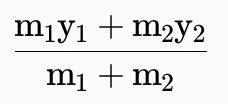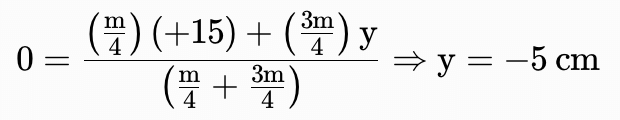## AFMC Mock Test Series

1 docs|30 tests
 Use Code STAYHOME200 and get INR 200 additional OFF Use Coupon Code
Information about Sample AFMC Physics Mock Test Page
In this test you can find the Exam questions for Sample AFMC Physics Mock Test solved & explained in the simplest way possible. Besides giving Questions and answers for Sample AFMC Physics Mock Test, EduRev gives you an ample number of Online tests for practice

## AFMC Mock Test Series

1 docs|30 tests

### How to Prepare for NEET

Read our guide to prepare for NEET which is created by Toppers & the best Teachers